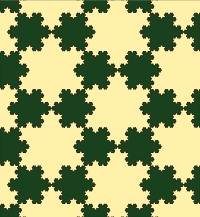# Koch SnowflakePhoto by David Eppstein

With the the official start of winter, many of us are going to be seeing more snow. But not all snowflakes are cold and wet. The Koch snowflake, first introduced by Swedish mathematician Niels Fabian Helge von Koch in his 1904 paper, is one of the earliest fractal curves to have been described. In his paper, von Koch used the Koch curve to illustrate that it is possible to have figures that are continuous everywhere but differentiable nowhere.

The Koch snowflake is constructed using a recursive algorithm. Starting with an equilateral triangle, each side is altered as follows:

1. Remove the inner third of the side.
2. Draw an equilateral triangle at the location where the piece of the side was removed, with the new triangle pointing away from the body of the original triangle.
3. Remove the line segment that is the base of the new triangle from Step 2.

The Koch snowflake is obtained as the limit of iterating these steps indefinitely. When von Koch first described this process, he used the example of a single straight line, which is known as the Koch curve. The Koch snowflake can thus be thought of as taking three Koch curves and putting them together.

The Koch snowflake has many interesting properties. It is an example of a figure that is self-similar, meaning that it looks the same on any scale. In addition, even though the Koch snowflake has an infinite perimeter, its area is finite. To see why this is the case, we first consider the number of sides at each iteration of the recursive algorithm above. For each iteration, one side of the figure becomes four sides in the following stage. Since we begin with three sides, the number of sides is given by$N_n = N_{n-1}* 4 = 3* 4^n$

for the$n$th iteration, starting at$n = 0$ for the original equilateral triangle. In every iteration, the length of a side is 1/3 the length of a side from the preceding stage. If we suppose that the original equilateral triangle had a side length of$s$, then the length of each side of the snowflake after$n$ iterations is$S_n = \dfrac{S_{n-1}}{3} = \dfrac{s}{3^n} ,$

and so the perimeter of the snowflake after$n$ iterations is$P_n = N_n* S_n = 3*s* \left( \dfrac{4}{3}\right)^n .$

Since the Koch snowflake is obtained by taking an infinite number of iterations, we see that the perimeter of the snowflake is$\displaystyle\lim_{n\to\infty} P_n = \lim_{n\to\infty} 3*s*\left( \dfrac{4}{3}\right)^n = \infty ,$

since$|\frac{4}{3}| > 1$. Thus, the Koch snowflake has an infinite perimeter. Now, we will look at the area of the snowflake. At each iteration, a new triangle is added onto each side from the previous iteration, so the number of new triangles added at iteration$n$ is$T_n = N_{n-1} = 3*4^{n-1} = \dfrac{3}{4}*4^n .$

The area of each new triangle added is one ninth of the area of each triangle added in the previous iteration, so the area of each triangle added at iteration$n$ is$A_n = \dfrac{A_{n-1}}{9} = \dfrac{A_0}{9^n} ,$

where $A_0$ is the area of the original equilateral triangle. The total new area added at iteration$n$ is therefore$B_n = T_n* A_n = \dfrac{3}{4}* \left( \dfrac{4}{9}\right)^n * A_0 .$

The total area of the snowflake after$n$ iterations is$\displaystyle a_n = A_0 + \sum_{k = 1}^{n} B_k ,$

which can be written as a geometric sum

$a_n = \dfrac{A_0}{5}\left( 8 – 3\left( \dfrac{4}{9}\right)^n \right).$

Again, since the Koch snowflake is obtained by taking an infinite number of iterations, we see that the area of the snowflake is

$\displaystyle \lim_{n\to\infty} a_n = \lim_{n\to\infty} \dfrac{A_0}{5}\left( 8 – 3\left( \dfrac{4}{9}\right)^n \right)= \dfrac{8}{5}* A_0 ,$

since$|\frac{4}{9}|< 1$. Therefore, the Koch snowflake has an infinite perimeter, but finite area.

Let it snow, let it snow, let it snow!

Sources:

Boccara, N.,SpringerLink (Online service). (2007). Essentials of mathematica: With applications to mathematics and physics. New York: Springer.